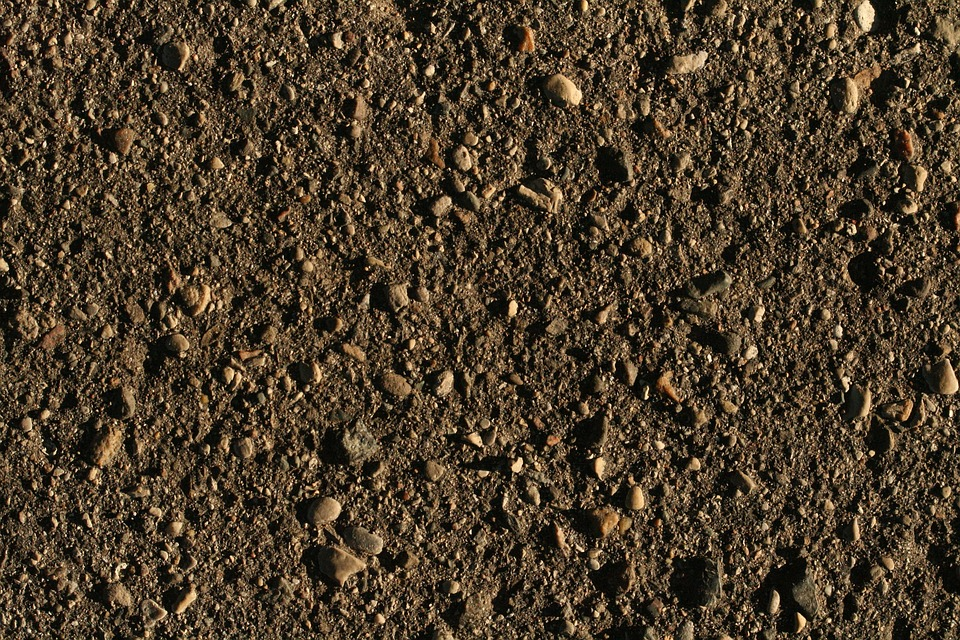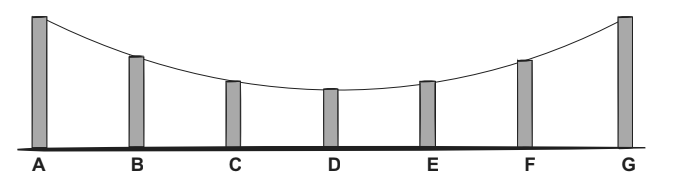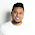## Tuesday, 3 July 2018

### Level 5 Extension

Level 5 Extension!

Two different shops advertise oranges for sale.Shop A =\$3.20 for 2kg
. Shop B= \$4.50 for 3kg

Which shop is giving the best buy for 7kg of oranges? Shop BTwo building suppliers advertise soil for sale.
Building Supplier A offers soil at \$130 per 2 cubic metres.
Building Supplier B offers topsoil at \$25 per 1⁄2 cubic metre.

Which supplier offers the best buy for 3 cubic metres of topsoil? Building Supplier B

INVESTIGATION Use any of the the symbols (, ), +, -, ×, or ÷ to make the expression below into the largest possible number. Each symbol can only be used once.
9 7 16 0.5

APPLICATIONThe above diagram is of a section of a bridge. A, B, C, D, E, F, G are vertical supports.
The vertical support A = 10 metres long.
ALSO:  length A = G, length B = F length C = E.

The length of B is 3/ 5 of A.- 6 m
The length of C is ⅘ of B- 4.8 m
The length of D is 9/10 of C - 0.48m
Calculate the total length of the supports. 12.28

FRACTIONS TO DECIMALS Change these fractions to decimals.
1/10  - 0.1                ⅜ - 0.375
3/10 - 0.3                   3/1000 - 0.003
⅖  - 0.4                             21/50 - 0.42
17/10 - 1.7                          8/20 - 0.4
⅛ - 0.125                                1 ¾ - 1.75

DECIMALS TO FRACTIONS Change these decimals to fractions.
0.4 - 4/10          0.0002 - 2/10000
0.314 - 314/100
0.5 - 5/10
0.175 - 175/1000
0.28 - 28/100

QUICK QUESTIONS Use any strategy. Don’t use a calculator.
1. 1.41 + 2.53 = 3.94
2. 25.4 - 24.1 = 1.3
3. 25 × 7 = 175
4. 2.5 × 7 =17.5
5. 24 ÷ 3 = 8
6. 2.4 ÷ 3 = 0.8
7. Round 3.156 to 1 DP. 3.2
8. -2 + 4 = 2
9. -2 - -3 =1
10. 3 - -4 + -5 = 2
11. 2.15 + 4.62 = 6.77
12. 7.53 - 7.39 = 0.14
13. 24 × 6 =79.44
14. 2.4 × 6 = 14.4
15. 141 ÷ 3 = 47
16. 14.1 ÷ 3 =4.7
17. Round 5.394 to 1 DP. 5.4
18. -12 + 3 = -9
19. -4 - -6 = 2

20. -8 + -2 - -6 = -4

#### 1 comment:

1.Keep up the good work.